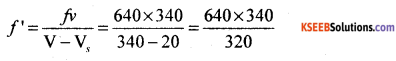# 1st PUC Physics Previous Year Question Paper March 2019 (North)

Students can Download 1st PUC Physics Previous Year Question Paper March 2019 (North), Karnataka 1st PUC Physics Model Question Papers with Answers helps you to revise the complete Karnataka State Board Syllabus and score more marks in your examinations.

## Karnataka 1st PUC Physics Previous Year Question Paper March 2019 (North)

Time: 3.15 Hours
Max Marks: 70

General Instructions:

1. All parts are compulsory.
2. Draw relevant figure / diagram wherever necessary.
3. Numerical problems should be solved with relevant formulae.

Part – A

I. Answer ALL the following questions: ( 10 × 1 = 10 )

Question 1.
How many kilograms is in one unified atomic mass unit?
1 amu = 1.66 × 10-27 kg.

Question 2.
State the law of triangle of addition of two vectors.
If two vectors $$\overrightarrow{\mathrm{A}}$$ and $$\overrightarrow{\mathrm{B}}$$ acting at a point are represented by the two sides of a triangle taken in an order then the closing side of the triangle represented in opposite order with respect to the other vectors, gives their resultant vector.

Question 3.
State Aristotle’s fallacy.
Aristotle assumed that force is required in order to set bodies in motion. However if a body is already in an uniform motion along a straight line, no external forces are required.

Question 4.
Name the type of energy stored in a stretched or compressed spring.
Potential Energy.Question 5.
Give an example for torque or moment of couple.
When two equal and opposite forces are applied at the two ends of the lid of the bottle, the lid experience the torque or rotational effect.

Question 6.
Write the aim of Cavendish experiment in gravitation.
Cavendish experiment determines the gravitational force of attraction between two ‘1 kg’ mass separated by ‘1 m’ apart.

Question 7.
How does strain depend on stress?
Strain is directly proportional to stress.
Strain ∝ Stress.

Question 8.
Mention the value of steam point of water in Fahrenheit scale.
Steam point = 100°C = 212 °F.

Question 9.
Which quantity is kept constant in adiabatic process?
In an adiabatic process, entropy oft he system will remain constant.Question 10.
The function y = log (ωt). The displacement y increases monotonically with time t, is it periodic function or non-periodic function.
Non-periodic function.

Part – B

II. Answer any FIVE of the following questions: ( 5 × 2 = 10 )

Question 11.
Name any two fundamental forces in nature.

1. Gravitational force
2. Electro – weak force
3. Strong nuclear force

Question 12.
Define accuracy in the measurement. How does accuracy depend on precision in the measurement.
Accuracy refers to finding of the result very close to expected result whereas precision is limited to the error in the instrument.Question 13.
Distinguish between speed and velocity.
Average Speed:

• The ratio of total distance traveled to the total time gives the average speed.
• Average speed is a scalar physical quantity.
• Average speed of a particle is finite; cannot be zero and always positive.
• Average speed is greater than the magnitude of average velocity.

Average Velocity:

• The ratio of net displacement of the particle to the total time taken gives the average velocity.
• Average velocity is a vector physical quantity.
• Average velocity of a particle may be zero, negative and positive.
• Average velocity (magnitude) is less than the speed of the particle.

Question 14.
Statement: Everybody continues to be in a state of rest or uniform motion along a straight line until an unbalanced force acts on it.
Explanation:

• Consider the mouth of a glass tumbler covered by a thin sheet of paper with a heavy coin on it. When the sheet of paper is pulled suddenly, the coin falls vertically down-wards into the glass tumbler due to inertia of position.
• Consider a book lying on a table. A force is required in order to cause any linear motion in it. In the absence of an external force, the book continues to occupy that position..

Question 15.
Mention the relation between linear momentum and angular momentum with usual meanings.
$$\vec{L}=\vec{r} \times \vec{p}$$ where $$\vec{p}$$ = linear momentum, $$\vec{L}$$ = angular momentum vector, $$\vec{r}$$ = radius or distance vector.

Question 16.
Write any two practical applications of Pascal’s law.
Hydraulic brakes, hydraulic press, hydraulic lifts, hydraulic jack, injection syringes.

Question 17.
Give any two assumptions of kinetic theory of gases.

1. Collisions of molecules on the walls are perfectly elastic.
2. The size of the molecules are neglected when compared to the volume of the container.
3. The contribution to the net pressure due to inter-molecular collisions is neglected.
4. The root mean square value of speed is the average of velocities taken in all the three directions.Question 18.
How does the period of oscillation of a pendulum depend on mass of the bob and length of the pendulum?
‘T’ is independent of mass and directly proportional to the square root of the length of the pendulum.
$$\mathrm{T} \propto \sqrt{\mathrm{L}}$$

Part – C

III. Answer any FIVE of the following questions : ( 5 × 3 = 15 )

Question 19. Deduce the expression for horizontal range of a projectile. For what angle of pro jection does horizontal range become maximum?
Expression for range of the projectile
We know that (v0 cosθ) remains constant.
Instantaneous distance along the horizontal is given by x = (v0 cosθ)t
for x = R (range of the projectile),
t = T= time of flight.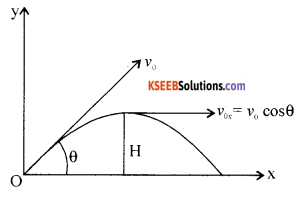Hence R= $$\left(v_{0} \cos \theta\right)\left(\frac{2 v_{0} \sin \theta}{g}\right)$$
Note: R = $$=\frac{2}{\mathrm{g}} v_{0 \mathrm{x}} v_{0 \mathrm{y}}$$
i.e., R = $$\frac{v_{0}^{2} \sin 2 \theta}{g}$$ where sin2θ = 2sinθ cosθ
Hence R ∝ v02 for the given angle of projection.Question 20.
Write any three laws of friction.

1. Limiting friction depends on the nature of the surfaces in contact but not on the surface area as long as normal reaction remains the same.
2. Force of friction always acts tangential to the surface and opposite the direction of motion.
3. The limiting friction is directly proportional to the normal reaction between two surfaces in contact.
4. The kinetic friction becomes constant, once there is a relative motion between the two surfaces.
5. μsk > μr where μs, μk and μr are coefficient of friction due to static friction, kinetic friction and rolling friction respectively.

Question 21.
Deduce the work-energy theorem for a constant force.
The difference of P.E. or K.E. required by a body at two different positions is equal to the amount of work done.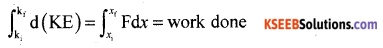i.e., W = Kf – Ki.

Question 22.
Deduce the equations of motion of centre of mass.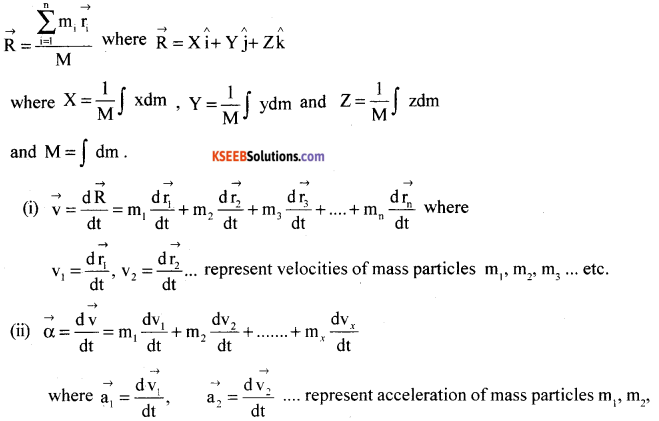Question 23.
Name the three types of moduli of elasticity.

1. Young’s Modulus
2. Bulk Modulus
3. Rigidity Modulus.Question 24.
What is capillary rise? Write the expression for height of capillary rise in the capillary tube with usual meanings.
The phenomenon of rise or fall of a liquid in a capillary tube is known as capillarity.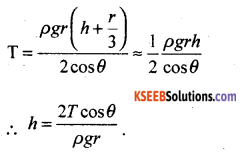Question 25.
Obtain an expression for thermal stress.
Y = $$\frac{\text { stress }}{\text { strain }}$$ so that stress = (Y) (strain)
i.e. stress = Y ∝ ∆θ
where stress represents thermal stress, Y – Young’s Modulus of elasticity.

Question 26.
Show that specific heat capacity of a solid is equal to three times that of Gas constant (C = 3R).
For solids U = 3RT.
At constant pressure, ∆Q = ∆U
∴C = $$\frac{\Delta \mathrm{Q}}{\Delta \mathrm{T}}$$ = 3R .

Part – D

IV. Answer any TWO of the following questions : ( 2 × 5 = 10 )

Question 27.
What is velocity – time graph. Deduce x = v0t +$$\frac{1}{2}$$at2 by using velocity-time graph.
A graph of velocity of a particle plotted along the Y-axis and time along the X-axis is known as v-t graph and the curve is known as velocity time curve.
Let ‘v0’ be the initial velocity of a particle. Let ‘a’ be the B uniform acceleration v – t graph gives a straight line with a constant slope, tanθ = m
i.e. a = $$\frac{v-v_{0}}{t}$$……..(1)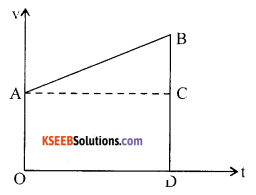From the figure OABD is a trapezium.
Area of trapezium OABD = Area of rectangle OACD + Area of triangle ACB
The second term on the right indicates the additional distance covered by the particle due to acceleration.
i.e., area of trapezium = (OA)(OD) + 1/2 (AC)(BC) = v0t + 1/2 t(v – v0) by using (1)
Area of trapezium = v0t + 1/2 at2
From the dimensional analysis. the right hand terms indicate the distance travelled. From the principle of homogeneity, the left hand side term should indicate the distance covered.
Hence area of the trapezium = x = v0t + 1/2 at2
For a uniform motion, a = 0 and x = v0t
For a particle starting from rest v0 = 0, x = 1/2 at2
in a vector form, $$\vec{x}=\vec{v}_{0} t+\frac{1}{2} \overrightarrow{a t}^{2}$$Question 28.
State and illustrate the law of conservation of linear momentum for any two colliding particles in a closed system.
Statement: In an isolated system of collision of bodies, the total linear momentum before impact is equal to the total linear momentum after impact.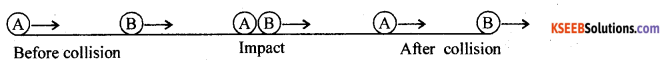Let m1 and m2 be the masses of two bodies moving along $$\vec{v}_{1 i}$$ and $$\vec{v}_{2 i}$$. Let $$\vec{v}_{1 f}$$ and be the $$\vec{v}_{2 f}$$ be final velocities after the impact.
At the time of impact the force of action acts on the body B and the force of reaction acts on A.
Applying Newton’s III law of motion
$$|Force of action on \mathrm{B}|=-| Force of reaction on \mathrm{A} |$$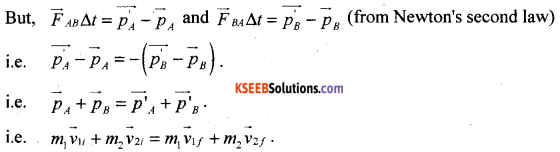This shows that the total final linear momentum of the isolated system equals its total initial momentum.

Question 29.
State and explain parallel axes and perpendicular axes theorems of moment of inertia.
Statement: The moment of inertia of a body about any axis is equal to the sum of the moment of inertia of the body about a parallel axis passing through the centre of mass and the product of mass of the body and square of the distance between the two parallel axes.
I = I0 + Md2.
Let ‘d’ be the distance between the two parallel axes. Let I be the moment of inertia about an axis passing through the centre of mass of a thin rod of length L.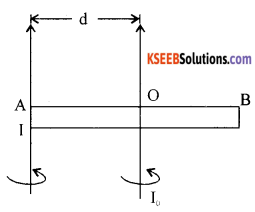We know that I0 = $$\frac{\mathrm{ML}^{2}}{12}$$ and d = $$\frac{\mathrm{L}}{2}$$
Hence moment of inertia at one end = $$\frac{M L^{2}}{12}+M\left(\frac{L}{2}\right)^{2}$$
I = $$\frac{M L^{2}}{4}\left[\frac{1}{3}+1\right]=\frac{M L^{2}}{3}$$

Statement : The moment of inertia about an axis perpendicular to two other axes acting in the same plane with their point of intersection being a point on it and the (third) axis passing through the common point, is equal to the sum of moments of inertia about the two axes.
e.g: Iz = Ix + Iy.
Let M be the mass of the disk of radius R.
M.I. about a point passing through the centre and perpendicular to the plane containing X and Y is I = $$\frac{M R^{2}}{2}$$
Since X and Y are in the same plane, Ix = Iy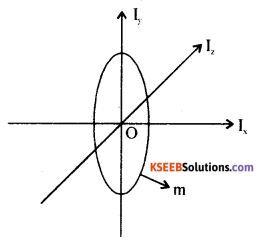∴ Iz = Ix + Iy becomes Iz = 2Ix
hence Ix = $$\frac{I_{Z}}{2}=\frac{M R^{2}}{4}$$
i.e. moment of inertia of a circular disc about the diameter $$\frac{I_{Z}}{2}=\frac{M R^{2}}{4}$$

V. Answer any TWO of the following questions: ( 2 × 5 = 10 )

Question 30.
State and explain the laws of thermal conductivity and hence mention the SI unit of coefficient of thermal conductivity.
The quantity of heat conducted during the steady state is directly proportional to the,
(i) Area of cross-section of a conductor.
(ii) Temperature difference between the two points or ends (θ1 – θ2).
(iii) Time of passage of heat (t) and inversely proportional to the thickness or distance between the two ends of the conductor (d).
i.e., Q = $$\frac{\mathrm{KA}\left(\theta_{1}-\theta_{2}\right) \mathrm{t}}{\mathrm{d}}$$
where ’K’ is thermal conductivity of the material of the conductor.
The SI unit of thermal conductivity is Wm-1 K-1.Question 31.
Deduce the expression for energy stored in a body executing simple harmonic motion.
We know that total energy of a particle executing SHM,
TE = KE + PE
where K.E= $$\frac{1}{2}$$ mv2
K.E = $$\frac{1}{2}$$mA2 cos2 (ωt + Φ)ω2
and P.E = $$\frac{1}{2}$$ ky2
i.e., P.E = $$\frac{1}{2}$$ mω2. A2sin2(ωt + Φ)
Hence T.E = $$\frac{1}{2}$$ mω2. A2 (cos2(cost + Φ) + sin2 (ωt + Φ))
or T.E = $$\frac{1}{2}$$ mω2. A2
Representing mω2 = k
we get TE = $$\frac{1}{2}$$ kA2
K.E of the particle=$$\frac{1}{2}$$ mω2. A2 – $$\frac{1}{2}$$ mω2. x2
= $$\frac{1}{2}$$ mω2(A2 -x2 )
where x = A cos (ωt + Φ)
Also K.E = $$\frac{1}{2}$$ mω2(A2)(1 – cos2(ωt + Φ))
i.e., K.E = $$\frac{1}{2}$$ mω2 A2sin2(ωt + Φ)
K(t) – K.E of a particle.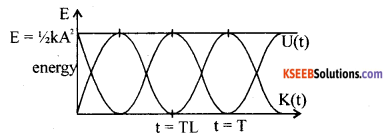U(t) of a particle.
T.E = K(t) + U(t) = $$\frac{1}{2}$$ kA2
where A – amplitude, k = mω2, m – mass of the particle
ω = angular frequency = $$\frac{2 \pi}{\mathrm{T}}$$
T = Period of SHM
TE = KE + PE = $$\frac{1}{2}$$mω2 A2 cos2 (ωt + Φ) + $$\frac{1}{2}$$ mω2 A2sin2(ωt + Φ) = $$\frac{1}{2}$$ mω2 A2

Question 32.
Discuss the mode of vibration of air columns in a closed pipe and hence define the fundamental frequency of vibration.
Let ‘L’ be the length of the closed pipe. A pipe with one end closed is known as a closed pipe system. Le V be the velocity of sound in air. Length of half segment = $$\frac{\lambda_{0}}{4}$$
i.e., L = $$\frac{\lambda_{0}}{4}$$ i.e., λ0 = 4L.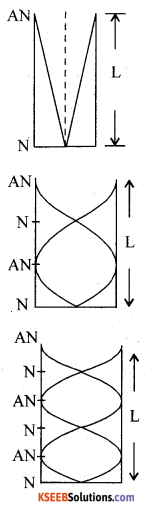The least mode of vibration is called fundamental mode i.e.,
fundamental frequency f0 = $$\frac{\mathbf{v}}{\lambda}=\frac{\mathbf{v}}{4 L}$$
In the second mode of vibration.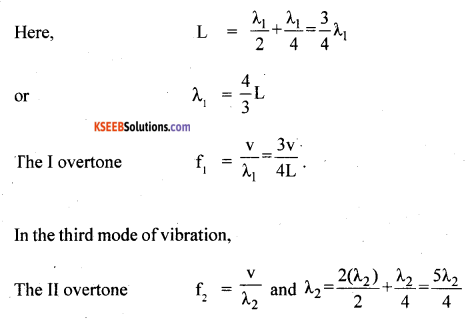i.e., f2 = $$\frac{5 v}{4 L}$$
Hence f0 : f1 : f2 :……..: fn : : 1 : 3 : 5 :………: (2n – 1)

VI. Answer any THREE of the following questions: ( 3 × 5 = 15 )

Question 33.
A stone of mass 0.25 kg tied to the end of a string is whirled round in a circle of radius 1.5 m with a speed of 40 revolutions per minute in a horizontal plane. What is the tension in the spring?
Given m = 0. 25 kg, R = 1.5 m, co = 40 rev. min-1
i.e., ω = $$\frac{40 \times 2 \pi}{60}$$ rad s-1
ω = $$\frac{40 \times 2 \pi}{60}$$
ω = $$\frac{4 \times 3.14}{3}$$ = 4.19 rad/s-1
Linear speed = v = rw = 1.5 × 4.19 = 6.285 ms-1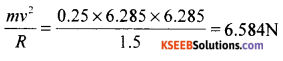T = 6.584 NQuestion 34.
A pump on the ground floor of a building can pump up water to fill a tank of volume 30 m3 in 15 minute. If the tank is 40 m above the ground and efficiency of the pump is 30%, how much of electrical power is consumed by the pump?
Given V = 30 m3, t = 15 min = 15 × 60 = 900s
h = 40 m, η = 30% , density ρ = 103 kg m-3, g = 10 ms-2
Potential Energy = mgh = Vρgh = 30 × 103 × 10 × 40
i.e. Work done = 12 × 106J.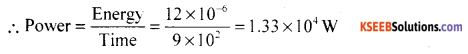True power required = $$\frac{100}{30}$$ × 1.33 × 104 = 4.433 × 104 W

Question 35.
If two spheres of equal masses with their centres 0.2 m apart attract each other with a force of 1 × 10-6 kg wt. What wrould be the value of their masses?
g = 9.8ms-2 and G = 6.67 × 10-11 Nm2kg-2.
Given r = 0.2 m,  F = 1 × 10-6 kgwt =1 × 10-6 × 9.8 = 9.8 × 106 N
G = 6.67 × 10-11 Nm2 kg-2, m1 = m2 = m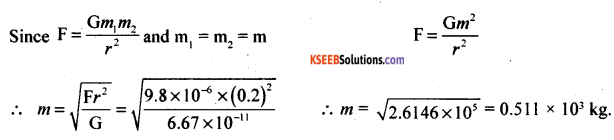i.e., mass used is 0.511 × 103 kg

Question 36.
The sink in Carnot’s heat engine is at 300K and the engine works at an efficiency 0.4. If the efficiency of the engine is to be increased to 0.5. Find by how many Kelvin the temperature of the source should be increased.
Given η = 0.4,  n1 = 0.5,  T = 300K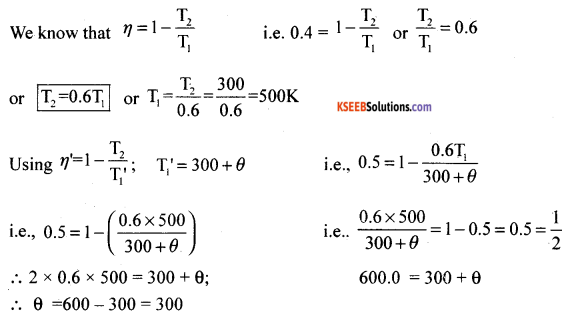The source temperature should be increased by 300 K.Question 37.
A train is moving at a speed of 72 kmph towards a station sounding a whistle, of frequency 640 Hz. What is the apparent frequency of the whistle as heard by a man standing on the platform when train approaches towards him.
Given : Speed of sound = 340 ms-1.